# gganimate with bitmap fonts

## Introduction

I finally got the time to play with the gganimate package by Thomas Lin Pederson.

The API is really well designed and Thomas has deconstructed the grammar of animation into a clean/orthogonal set of functions.

I put together the little animation shown below, and this is a short guide on how I got there.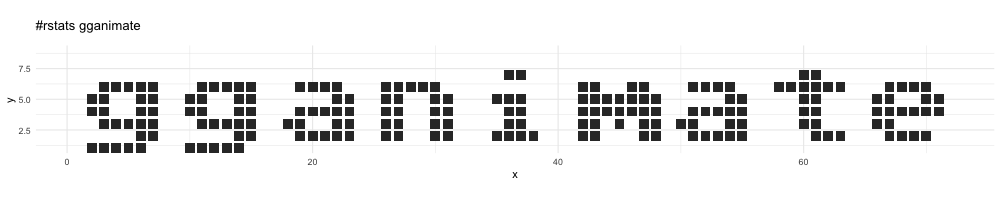## Bitmap fonts

In a bitmap font, a character is defined by a binary matrix - where the matrix is 1 the underlying pixel is turned on, otherwise it is turned off.

An example matrix of an 8x8 binary matrix to define the letter a is shown below:

     [,1] [,2] [,3] [,4] [,5] [,6] [,7] [,8]
[1,]    0    0    0    0    0    0    0    0
[2,]    0    0    0    0    0    0    0    0
[3,]    0    0    1    1    1    1    0    0
[4,]    0    0    0    0    0    1    1    0
[5,]    0    0    1    1    1    1    1    0
[6,]    0    1    1    0    0    1    1    0
[7,]    0    0    1    1    1    1    1    0
[8,]    0    0    0    0    0    0    0    0

This matrix can be easily plotted with the raster package

withr::with_package('raster', {
plot(raster(char_matrix$a), col=c(1,0), legend=FALSE, ann=FALSE, axes=FALSE) })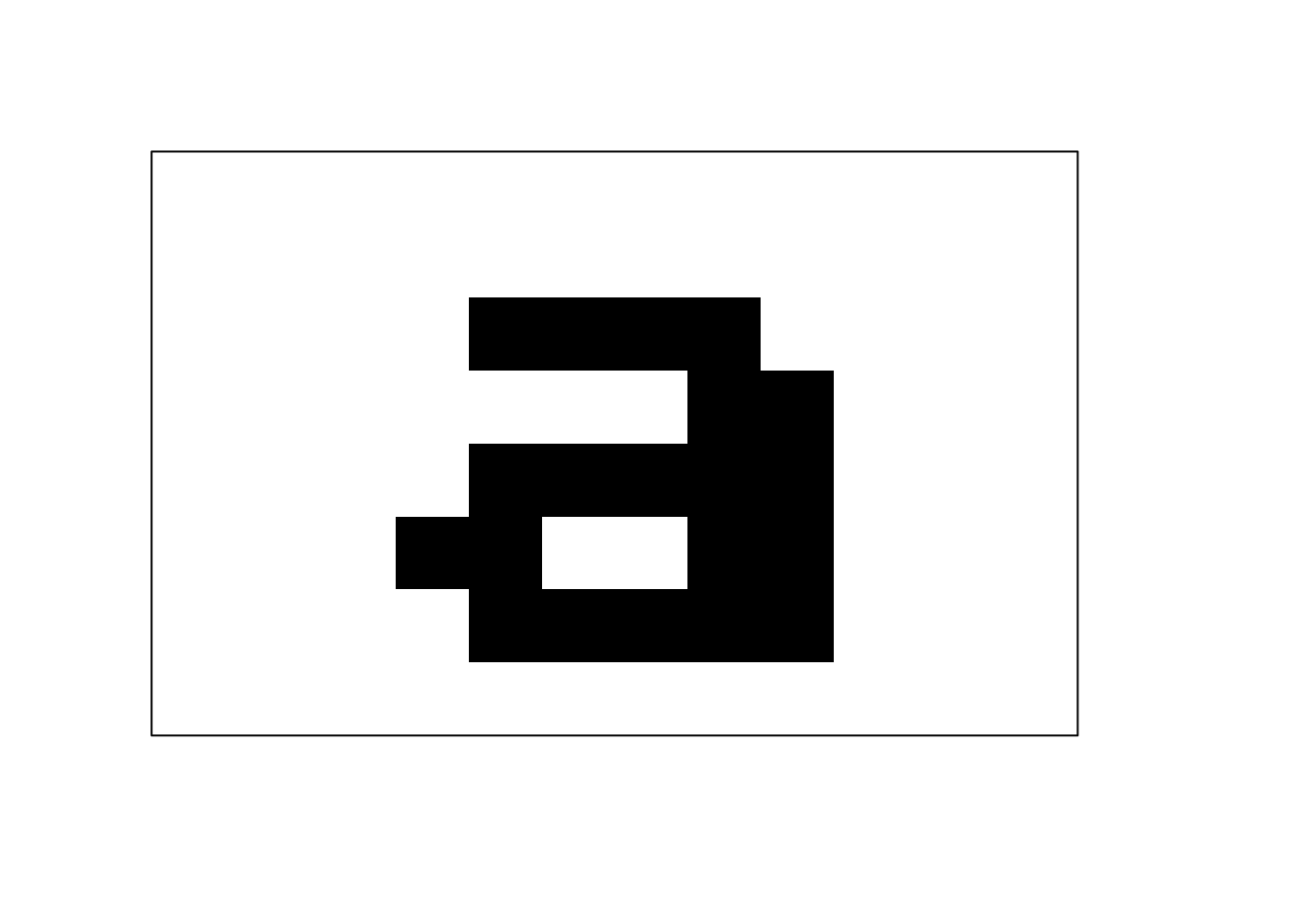Rather than individually creating bitmap font matrices, I’m actually reading in bitmap font files from disk. I haven’t packaged that code up yet, but hopefully will do so soon. For this post I’ll just use some manually created character data stored in char_df. ## Rendering a bitmap font character with ggplot2 To work with ggplot2, the matrix for an 8x8 character is turned into a data.frame. The data.frame is the x and y co-ordinates of all the locations of 1 in the bitmap matrix. Here is a data.frame for the same a, now ready for ggplot2.  x y 1 2 5 2 3 5 3 4 5 4 5 5 5 5 4 6 6 4 7 2 3 8 3 3 9 4 3 10 5 3 11 6 3 12 1 2 13 2 2 14 5 2 15 6 2 16 2 1 17 3 1 18 4 1 19 5 1 20 6 1 This data.frame is plotted using ggplot2::geom_tile() ggplot(char_df$a, aes(x, y)) +
geom_tile() +
coord_equal() +
theme_minimal()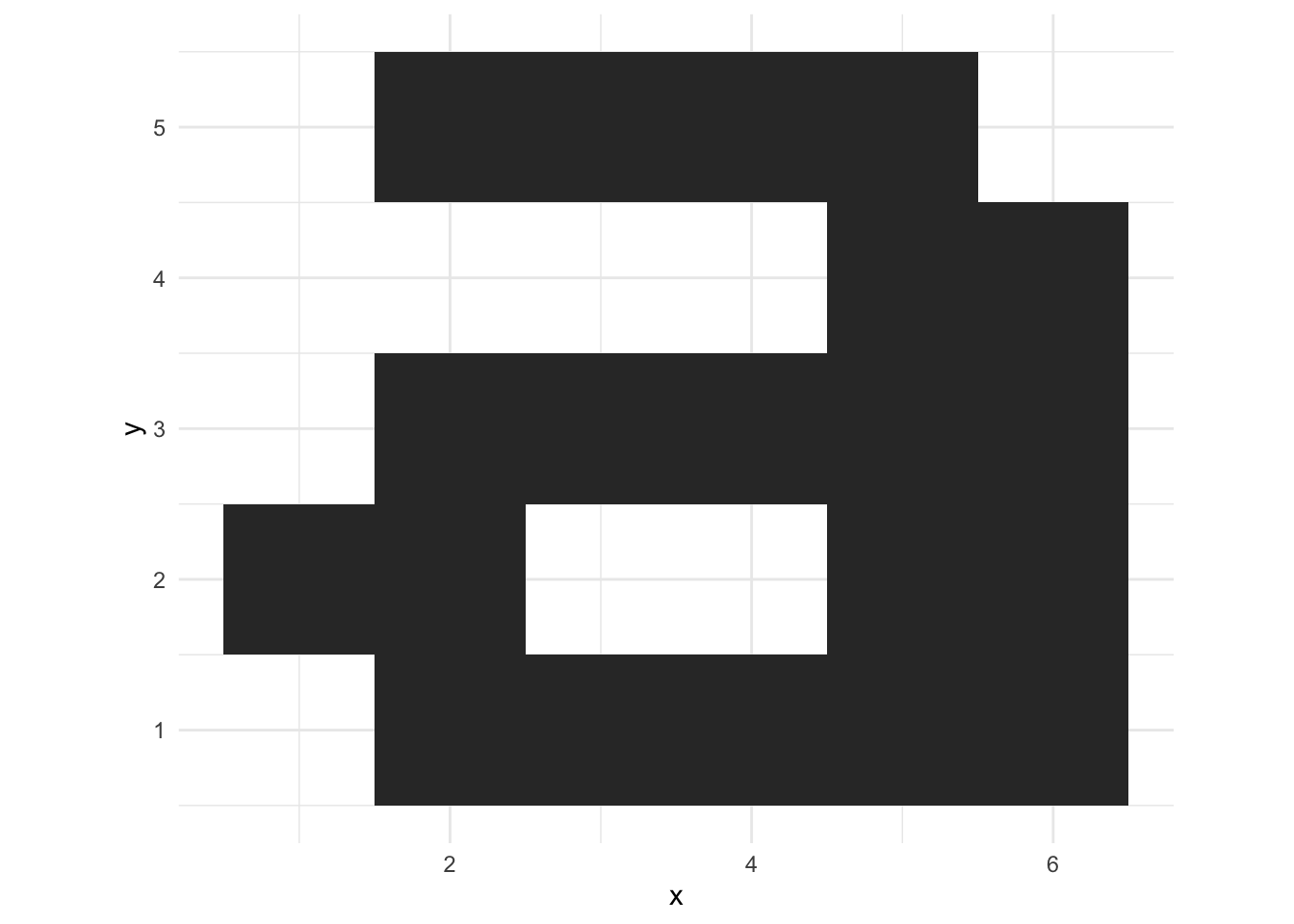The tile size is set a bit smaller than 1 to get the retro pixel look:

ggplot(char_df$a, aes(x, y)) + geom_tile(width=0.9, height=0.9) + coord_equal() + theme_minimal()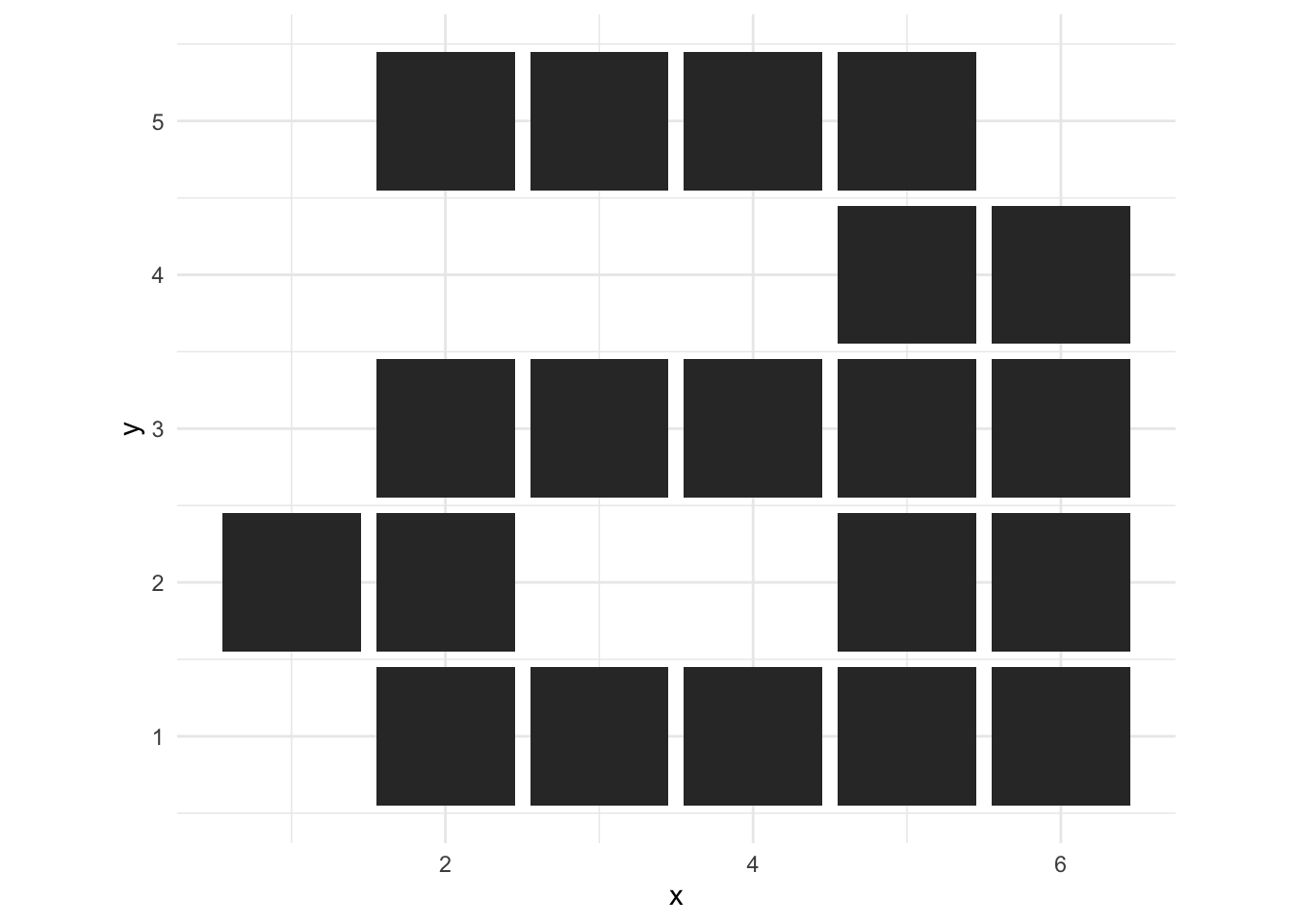## Preparing a data.frame for gganimate The data.frame for gganimate is going to consist of 3 characters (a, b, c), displayed one-at-a-time. • Create a data.frame for each bitmap character • Give each character an idx which will let gganimate control when it is displayed • Stack them all into a single data.frame with dplyr::bind_rows() plot_df <- dplyr::bind_rows( char_df$a %>% mutate(idx = 1),
char_df$b %>% mutate(idx = 2), char_df$c %>% mutate(idx = 3)
)

Plotting without gganimate results in the 3 letters being superimposed. Not really very useful, but a stepping stone to the actual animation.

p <- ggplot(plot_df, aes(x, y)) +
geom_tile(width=0.9, height=0.9) +
coord_equal() +
theme_minimal()

p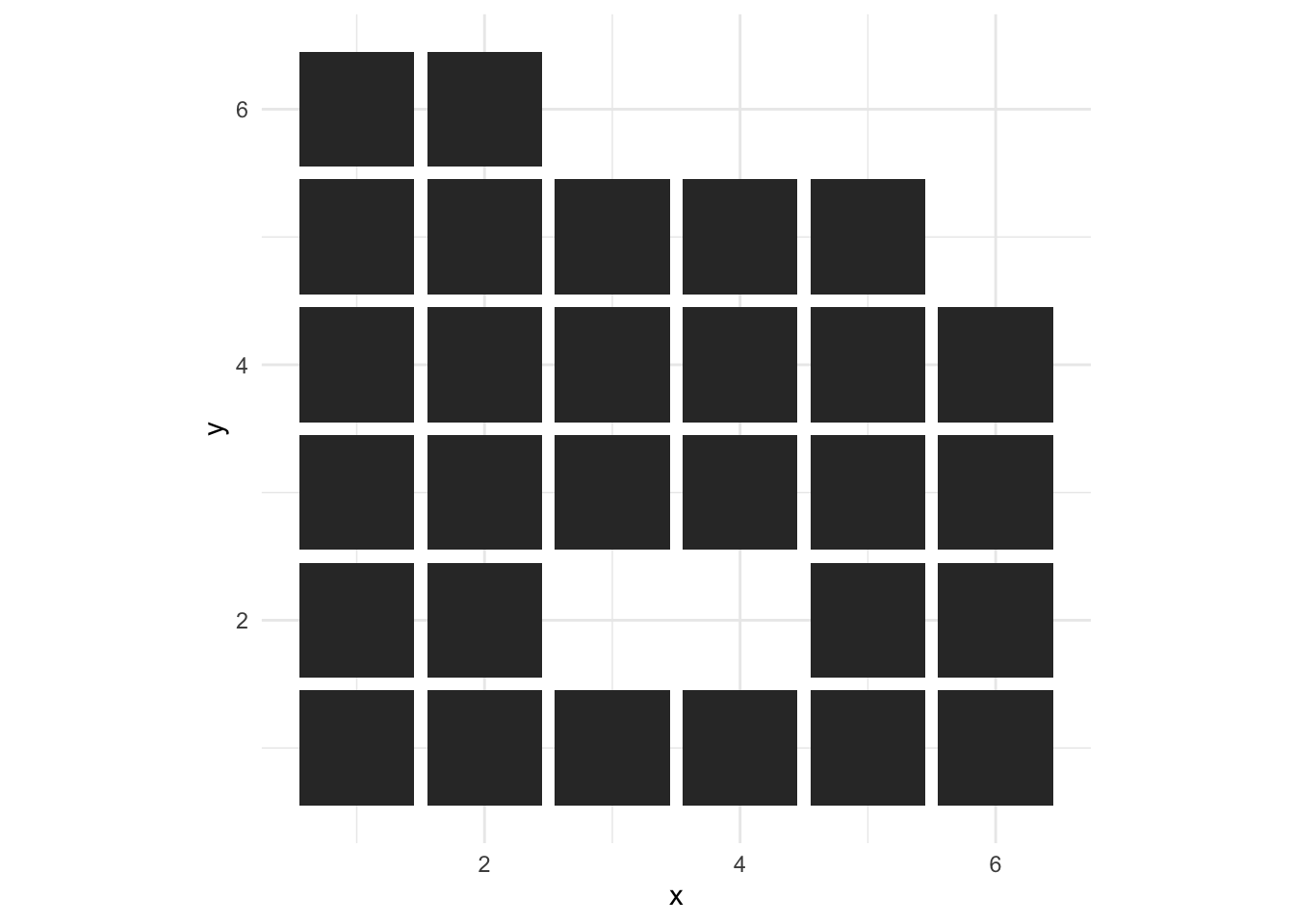Below I facet by idx to separate out each character in space. gganimate will use the idx column to separate the characters in time using transition_states().

p +
facet_wrap(~idx) +
theme_bw()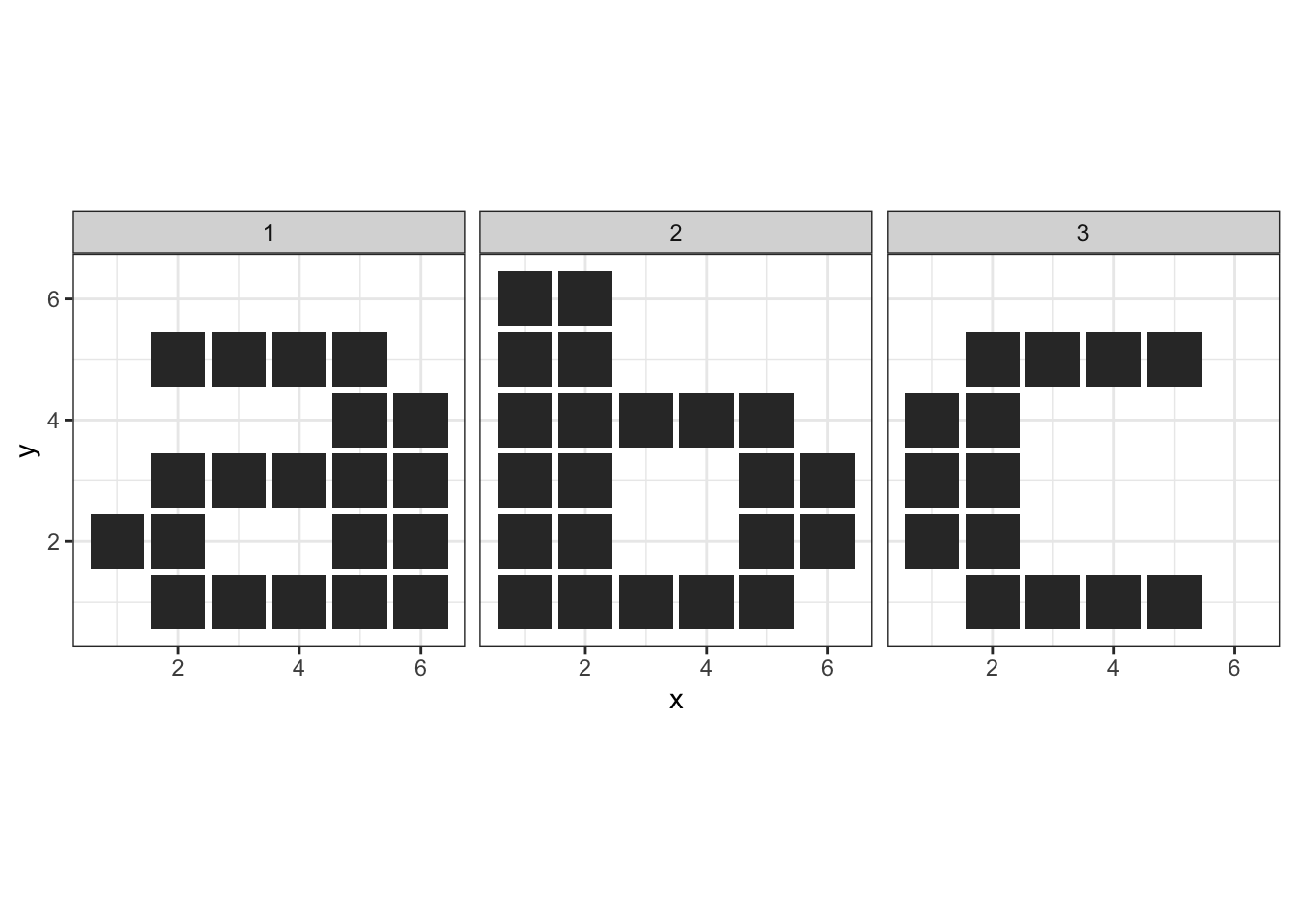## Plotting with gganimate - facetting in time!

gganimate takes a standard ggplot and separates out the individual states (i.e. the idx variable) by rendering them at different times.

By analogy:

• ggplot2::facet_wrap() renders the data at different locations in space dependent upon the facetting variable
• gganimate::transition_states() renders the data at different times dependent upon the states variable.
panim <- p +
transition_states(
states            = idx, # variable in data
transition_length = 1,   # all states display for 1 time unit
state_length      = 1    # all transitions take 1 time unit
) +
enter_fade() +             # How new blocks appear
exit_fade() +              # How blocks disappear
ease_aes('sine-in-out')    # Tweening movement

panim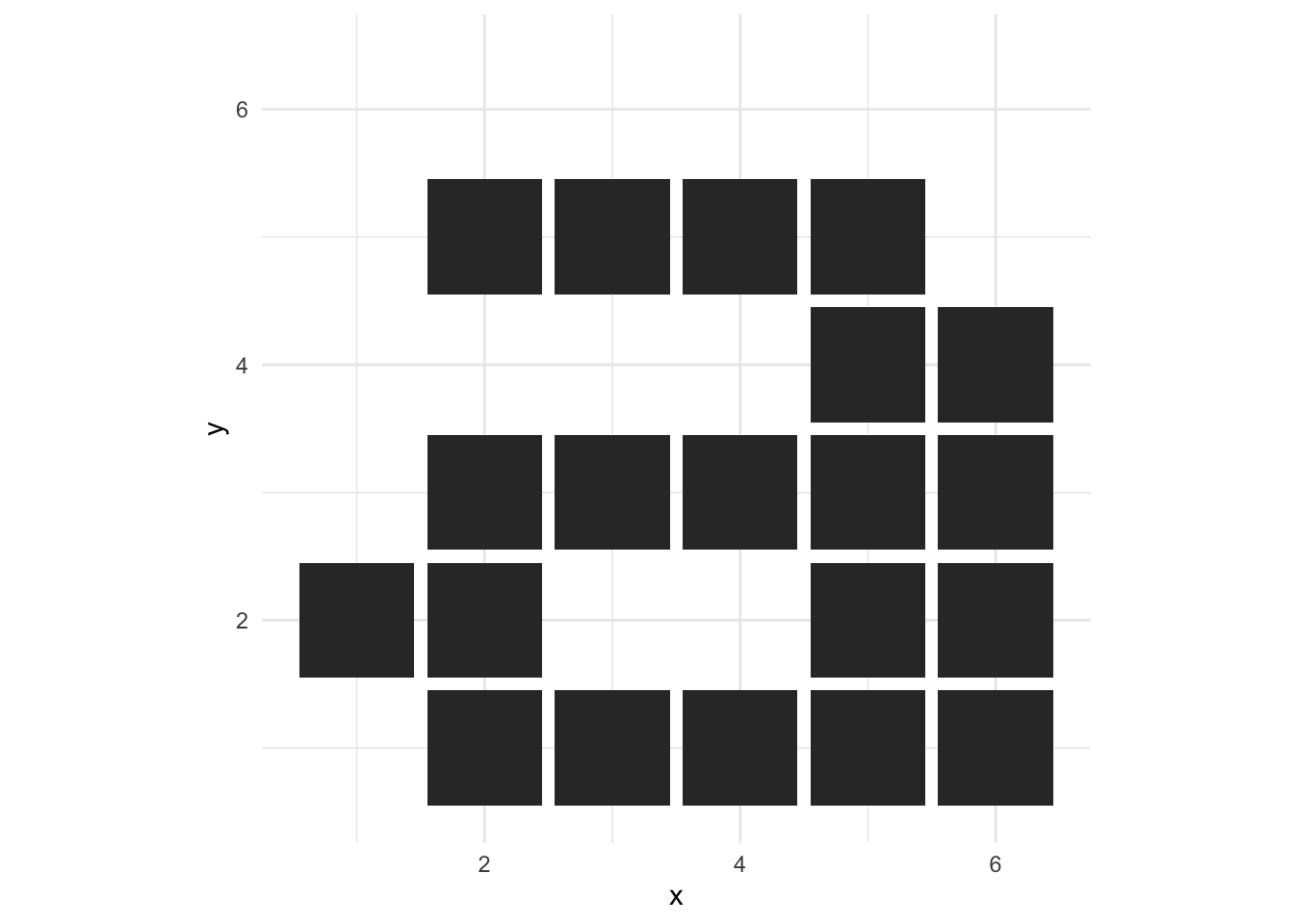## Controlling the animation length and image size

By default, the animation is scaled into 100 frames of PNG output at 10 frames-per-second. These png files are then rendered to an animated gif using gifski.

By default the individual images are rendered using R’s built-in png() which has a default output size of 480x480 pixels.

To render the gif differently (e.g. shorter duration, faster playback, smaller image size), you can call animate() directly on the plot object

animate(panim, fps=20, nframes=50, width=200, height=200)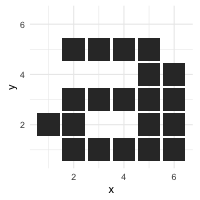## To Do

• Package up the code for parsing bitmap font files